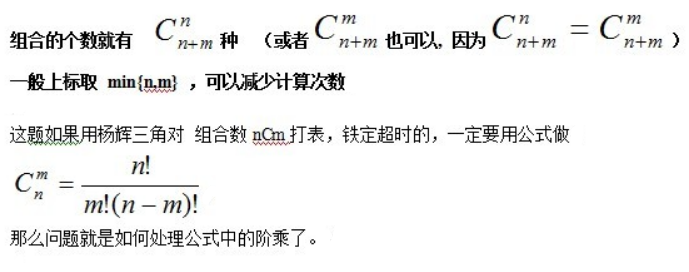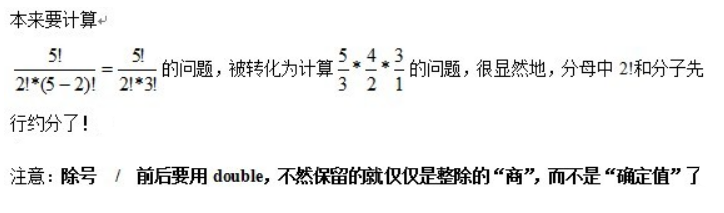# POJ 1942 - Paths on a Grid

## 解题思路

• 那么可以这样走： 上上上上上右右右右
• 又可以这样走： 上右上右上右上右上• （1）传统意义上的直接递归，n的规模最多到20+，太小了，在本题不适用，而且非常慢
• （2）稍快一点的算法，就是利用log()化乘为加，n的规模虽然扩展到1000+，但是由于要用三重循环，一旦n规模变得更大，耗时就会非常之严重,时间复杂度达到 `O(n*m*(n-m))`，本题规定了n，m用unsigned int32类型，就是说n,m的规模达到了21E以上，铁定TLE的。而且就算抛开时间不算，还存在一个致命的问题，就是精度损失随着n的增加会变得非常严重。
因为n有多大，就要进行n次对数运算，n规模一旦过大，就会丢失得非常严重了。所以这种方法是绝对不可取的，因为中途的精度丢失不是简单的四舍五入可以挽回的。
• （3）拆分阶乘，逐项相除，再乘以前面所有项之积。这种方法用一个循环就OK了，时间复杂度只有 O(n-m)，非常可观。

``````double cnm=1.0;
while(b>0)
cnm*=(double)(a- -)/(double)(b- -);``````## 解题方法一：四舍五入+强制类型转换

``````/*强制类型转换输出*/

//Memory Time
//220K   0MS

#include<iostream>
#include<math.h>
using namespace std;

/*Compute (n+m)C min{n,m}*/

unsigned comp(unsigned n,unsigned m)
{
unsigned a=m+n;
unsigned b=(m<n?m:n);
double cnm=1.0;
while(b>0)
cnm*=(double)(a--)/(double)(b--);

cnm+=0.5;      //double转unsigned会强制截断小数，必须先四舍五入
return (unsigned)cnm;
}

int main(void)
{
unsigned m,n;
while(true)
{
cin>>m>>n;
if(!m && !n)//承认这题的猥琐吧！竟然有其中一边为0的矩阵，一定要&&，用||会WA
break;

cout<<comp(n,m)<<endl;
}
return 0;
}``````

## 解题方法二：setprecision()函数+自定义精度输出

``````/*自定义精度输出*/

//Memory Time
//220K   0MS

#include<iostream>
#include<math.h>
#include<iomanip>
using namespace std;

/*Compute (n+m)C min{n,m}*/

double comp(unsigned n,unsigned m)
{
unsigned a=m+n;
unsigned b=(m<n?m:n);
double cnm=1.0;
while(b>0)
cnm*=(double)(a--)/(double)(b--);

return cnm;
}

int main(void)
{
unsigned m,n;
while(true)
{
cin>>m>>n;
if(!m && !n)
break;

cout<<fixed<<setprecision(0)<<comp(n,m)<<endl;
//fixed是为了固定小数位数
//setprecision()函数是会自动四舍五入的，所以不用像强制类型转换那样预先+0.5
}
return 0;
}``````

目录